# 20 Normal Distributions

The normal distribution, recognized for its canonical bell-shaped density function, is the single most important continuous probability distribution for statistical inference and theory. The reason that the normal distribution has such importance is its role the central limit theorem, which states, roughly, that the distribution of sums of independent and identically distributed random variables can be well approximated by normal distributions under fairly general conditions when the sample sizes are sufficiently large. A precise statement of the central limit theorem describes a limiting behavior, but the practical importance is that for many distributions and reasonable sample sizes, the normal distribution approximation can be very accurate. There are extensions to the central limit theorem for cases where the independence and identical distribution conditions may be relaxed to various forms of weak dependence and some differences in distribution, but these considerations are not important for this discussion.

Many real continuous random variables have distributions that are well approximated by normal distributions. Some random variables whose distributions are not well fit by a normal distribution might be transformed, for example by taking logarithms, to obtain a better approximation. More importantly, even when variables themselves are not well approximated, many numerical summaries of moderately sized or larger random samples can be approximated well.

The remainder of this chapter introduces the normal distribution and R commands to work with it.

## 20.1 Parameters

Each normal distribution is described fully by two parameters: the mean ($$\mu$$) and standard deviation ($$\sigma$$), using conventional notation. The mean is the weighted average of the possible values of the random variable, weighted by the probability density of each value. The standard deviation is the square root of the variance. The density is centered at the mean and the mean is the balancing point. The standard deviation is a measure of scale.

If a random variable $$X$$ has a normal distribution with mean $$\mu$$ and standard deviation $$\sigma$$, we often denote this $X \sim \text{Normal}(\mu, \sigma^2)$ where $$\sigma^2$$ is the variance. Some texts will label the distribution with the standard deviation instead of the variance.

## 20.2 Normal Probability Density

The formula for the normal density is $f(x) = \frac{1}{\sigma\sqrt{2\pi}} \mathrm{e}^{- \frac{1}{2}\left( \frac{x - \mu}{\sigma} \right)^2 }$

A graph of the density shows the bell-shaped curve.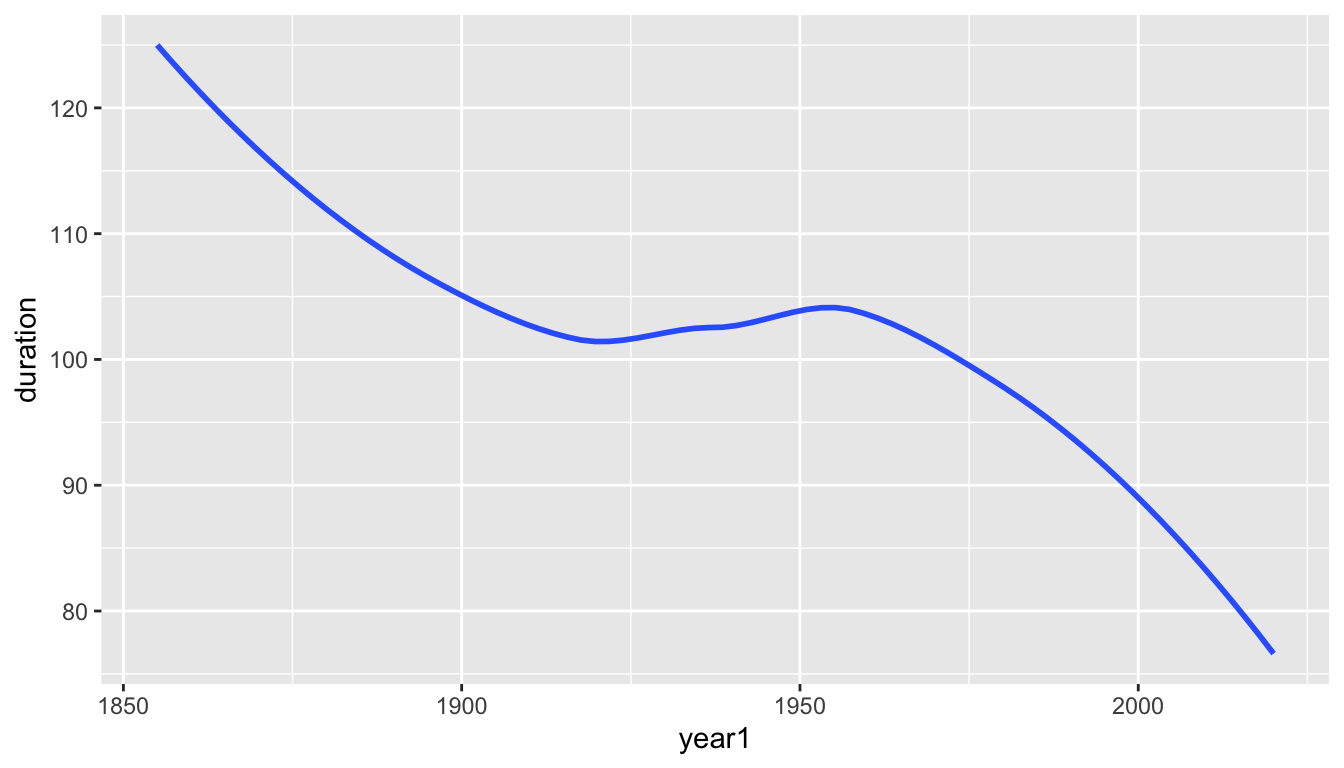• Every normal density has exactly the same shape.
• The density curve is symmetric around the mean
• The median is equal to the mean
• The locations one standard deviation below and above the mean are at the points of inflection where the slopes of tangent lines to the curve are steepest.
• The total area under the density curve is equal to one, as it is for every probability density.
• Probabilities are associated with areas under the density curve.

When multiple normal densities are drawn on the same axis, you can observe effects of modifying parameter values. The next plots displays densities from the following three normal distributions:

• Purple: $$\text{Normal}(0,1)$$
• Green: $$\text{Normal}(1,1)$$ (shifted right of the purple density)
• Yellow: $$\text{Normal}(0,2)$$ (more spread out than the purple density)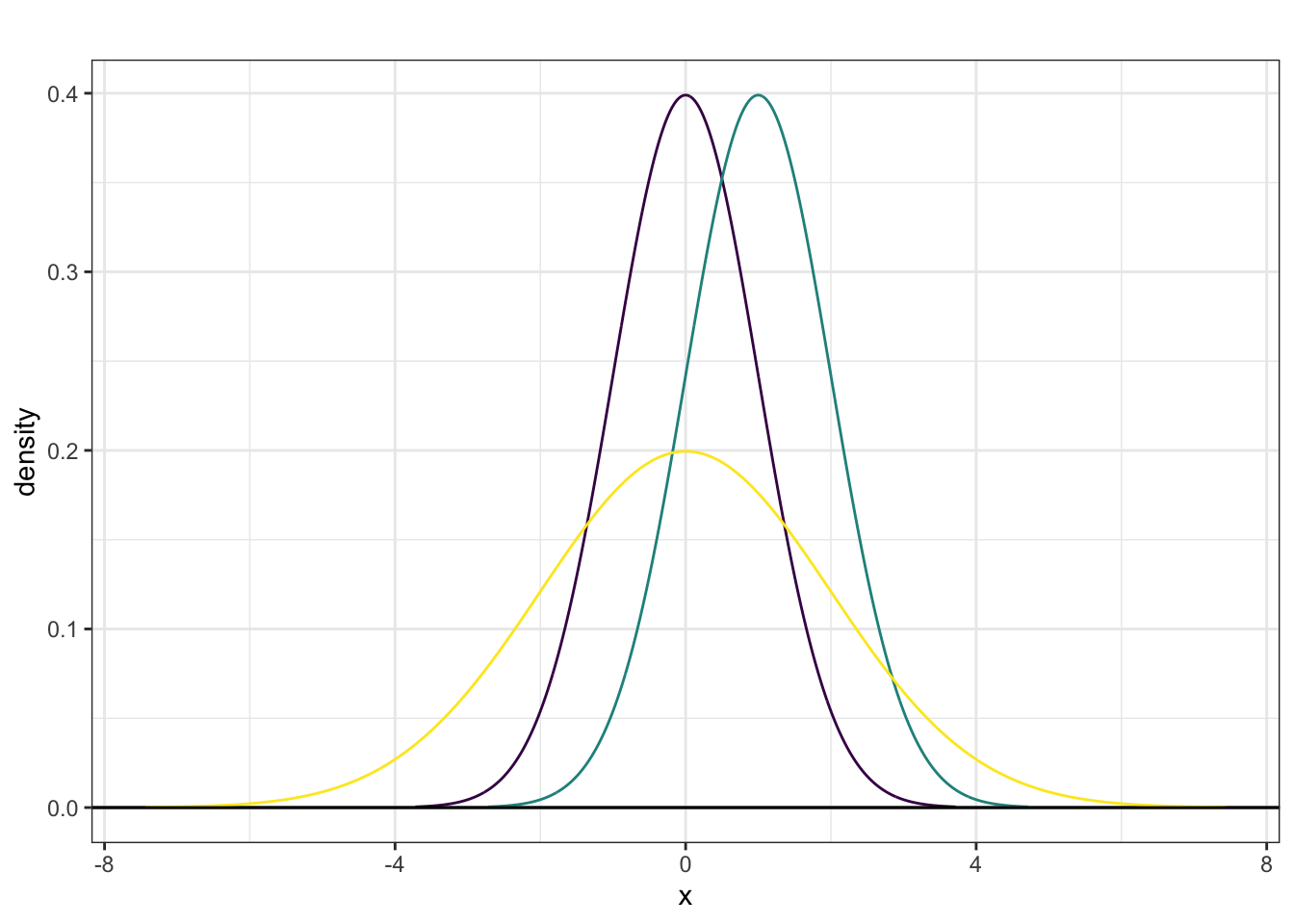## 20.3 Standard Normal Density

The standard normal density has mean $$\mu = 0$$ and standard deviation $$\sigma = 1$$.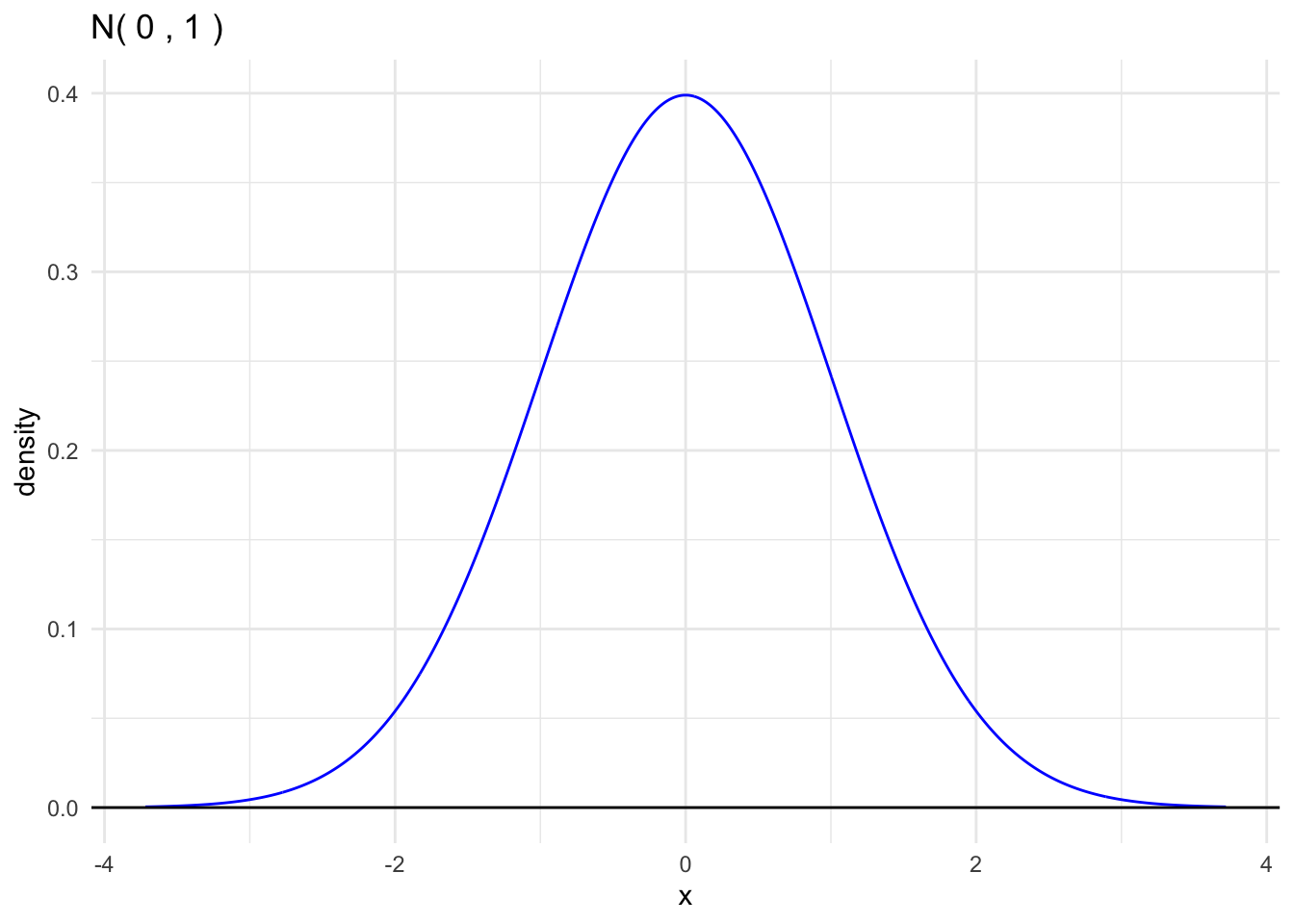We conventionally use the symbol $$Z$$ for a standard normal random variable. $Z \sim \text{Normal(0,1)}$

Every normal random variable may be converted to a standard normal random variable by a linear transformation: substract the mean to center at zero and divide by the standard deviation to rescale. $Z = \frac{X - \mu}{\sigma}$

Every normal random variable may be created by rescaling and recentering a standard normal random variable. $X = \mu + \sigma Z$

## 20.4 Benchmark Normal Probabilities

Every normal random variable has the following benchmark areas:

• The area within one standard deviation of the mean (between $$\mu - \sigma$$ and $$\mu + \sigma$$) is approximately 68%.
• The area within two standard deviations of the mean (between $$\mu - 2\sigma$$ and $$\mu + 2\sigma$$) is approximately 95%.
• The area within three standard deviations of the mean (between $$\mu - 3\sigma$$ and $$\mu + 3\sigma$$) is approximately 99.7%.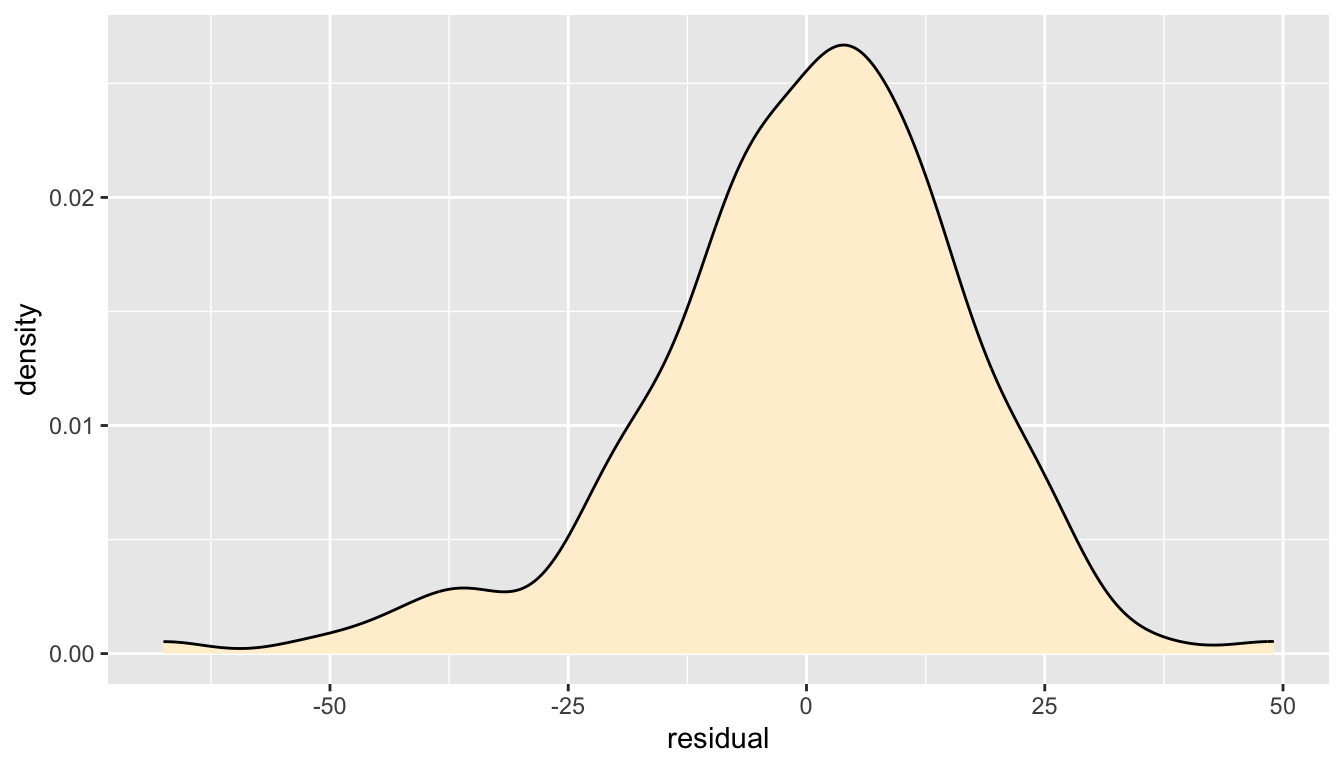These benchmarks are easily extended to tail areas.

• The area in the tail to the left (right) of $$\mu - \sigma$$ ($$\mu + \sigma$$) is about 16%.
• The area in the tail to the left (right) of $$\mu - 2\sigma$$ ($$\mu + 2\sigma$$) is about 2.5%.

## 20.5 Normal CDF

For any probability distribution, the cumulative distribution function (cdf) returns the probability that the random variable is less than or equal to the value. $F(x) = \mathsf{P}(X \le x)$

Note:

• For a continuous distribution like the normal, it does not matter if we use $$<$$ or $$\le$$ because the probability of being exactly at one value is zero (the area of a line segment is zero).
• However, the distinction matters for discrete random variables. For a binomial random variable, $$\mathsf{P}(X < 3)$$ and $$\mathsf{P}(X \le 3)$$ differ by $$\mathsf{P}(X = 3)$$.

For a normal distribution, this is the area to the left of $$x$$ under the corresponding normal density.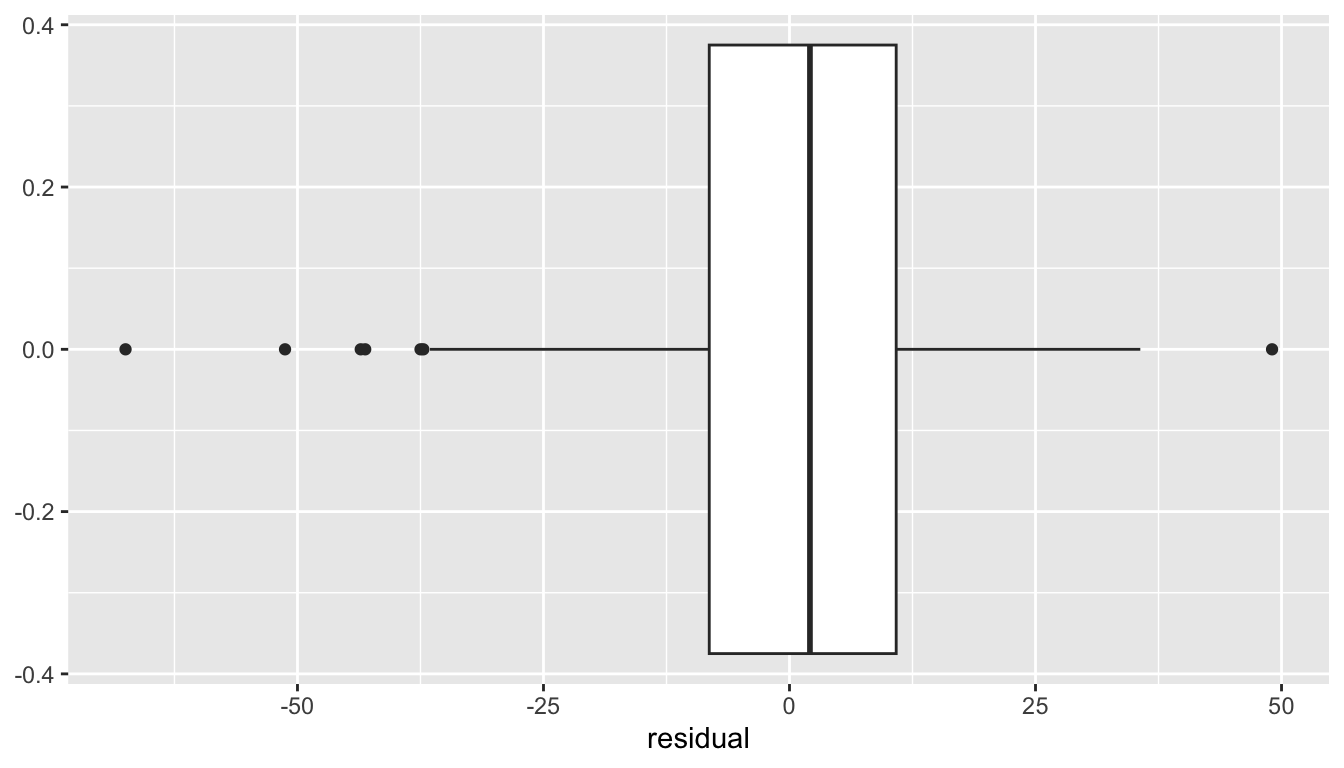For the standard normal curve, we use the special symbols $$\phi(z)$$ for the density and $$\Phi(z)$$ for the cdf:

$\phi(z) = \frac{1}{\sqrt{2\pi}} \mathrm{e}^{- \frac{z^2}{2}}$

Using notation from calculus, $\Phi(z) = \int_{-\infty}^z \phi(t)\, \mathrm{d}t$

Areas for any intervals are easily calculated in reference to the cdf.

• left tail: $$\mathsf{P}(Z < z) = \Phi(z)$$
• right tail: $$\mathsf{P}(Z > z) = 1 - \Phi(z)$$
• finite interval: $$\mathsf{P}(a < Z < b) = \Phi(b) - \Phi(a)$$
• outer area: $$\mathsf{P}(|Z| > a) = 2\Phi(-a)$$ is $$a>0$$ and $$1$$ is $$a < 0$$.

Areas for an arbitrary normal random variable are equivalent to a corresponding area under the standard normal curve, using the standardization change of variable.

If $$X \sim \text{Normal}(\mu, \sigma^2)$$, then

$\mathsf{P}(X \le x) = \mathsf{P}\left(\frac{X - \mu}{\sigma} < \frac{x - \mu}{\sigma}\right) = \mathsf{P}\left(Z \le \frac{x - \mu}{\sigma}\right)$

## 20.6 Central Limit Theorem

The random variables $$X_1, \ldots, X_n$$ are independent and drawn from a distribution $$F$$ where $$\mathsf{E}(X_i) = \mu$$ and $$\mathsf{Var}(X_i) = \sigma^2$$. The sample mean is a random variable defined as $\bar{X} = \frac{ \sum_{i=1^n} X_i }{ n }$ Then:

1. $$\mathsf{E}(\bar{X}) = \mu$$
2. $$\mathsf{Var}(\bar{X}) = \frac{\sigma^2}{n}$$
3. If $$n$$ is large enough, then the distribution of $$\bar{X}$$ is approximately normal.